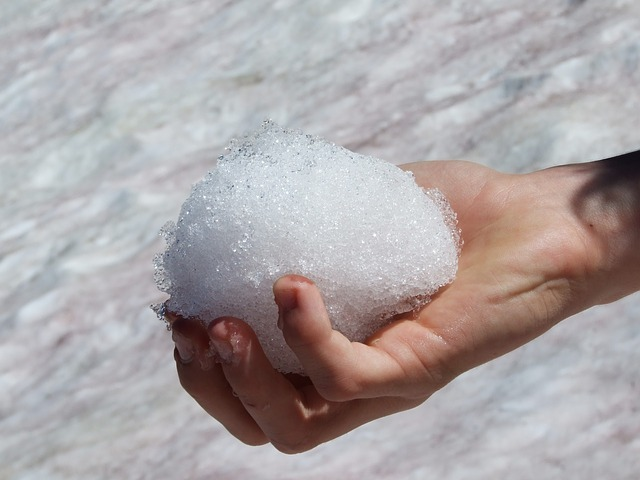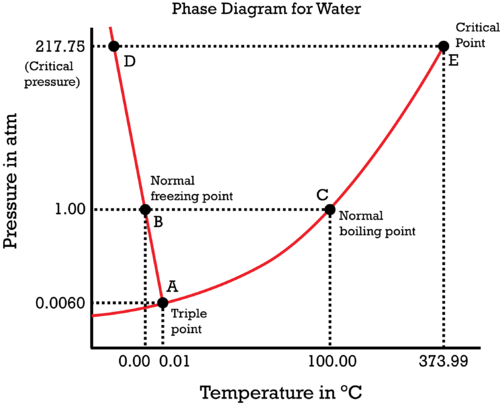## Phase Diagram for Water

### Learning Objectives

• Describe the phase diagram for water.
• Define critical temperature.
• Define critical pressure.

### Examples

#### How is it possible to create snowballs?

You need a special snow to make the best snowballs. This snow needs to be a little wet so the particles will stick together. Dry snow can be tightly pressed and will form snowballs because the higher pressure causes the snowflakes to melt somewhat. However, when you release the pressure, the snow goes back to a more solid form and the flakes no longer stick together.### Phase Diagram for Water

Water is a unique substance in many ways. One of these special properties is the fact that solid water (ice) is less dense than liquid water just above the freezing point. The phase diagram for water is shown in the Figure below .Figure 13.26

Phase diagram for water.

Notice one key difference between the general phase diagram and the phase diagram for water. In water’s diagram, the slope of the line between the solid and liquid states is negative rather than positive. The reason is that water is an unusual substance in that its solid state is less dense than the liquid state. Ice floats in liquid water. Therefore, a pressure change has the opposite effect on those two phases. If ice is relatively near its melting point, it can be changed into liquid water by the application of pressure. The water molecules are actually closer together in the liquid phase than they are in the solid phase.

Refer again to water’s phase diagram ( Figure above ). Notice point E, labeled the critical point . What does that mean? At 373.99°C, particles of water in the gas phase are moving very, very rapidly. At any temperature higher than that, the gas phase cannot be made to liquefy, no matter how much pressure is applied to the gas. The critical pressure (P c ) is the pressure that must be applied to the gas at the critical temperature in order to turn it into a liquid. For water, the critical pressure is very high, 217.75 atm. The critical point is the intersection point of the critical temperature and the critical pressure.

### Key Takeaways

#### Summary

• Solid water is less dense than liquid water just above the freezing point.
• The critical temperature (T c ) of a substance is the highest temperature at which the substance can possibly exist as a liquid.
• The critical pressure (P c ) is the pressure that must be applied to the gas at the critical temperature in order to turn it into a liquid.
• The critical point is the intersection point of the critical temperature and the critical pressure.

### Exercises

#### Practice

Questions

Read the section of the material at the link below that deals specifically with water:

http://www.chemguide.co.uk/physical/phaseeqia/phasediags.html

1. Why does the melting point of ice get lower at higher pressures?
2. Can you compress steam to form a liquid if the temperature is above 374°C?
3. What is the critical pressure of water?

### Exercises

#### Review

Questions

1. What happens to solid ice under high pressure near the freezing point?
2. What is the critical temperature?
3. What is the critical pressure?

## Glossary

• critical point: The intersection point of the critical temperature and the critical pressure.
• critical pressure: The pressure that must be applied to the gas at the critical temperature in order to turn it into a liquid.
• critical temperature: The highest temperature at which the substance can possibly exist as a liquid.